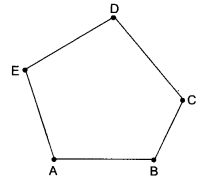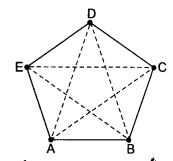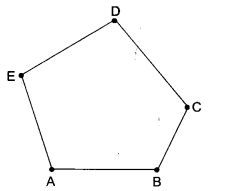# CBSEtips.in

## Sunday 28 February 2021

### CBSE Class 6 Maths - MCQ and Online Tests- Unit 4 - Basic Geometrical Ideas

#### CBSE Class 6 Maths – MCQ and Online Tests – Unit 4 – Basic Geometrical Ideas

Every year CBSE students attend Annual Assessment exams for 6,7,8,9,11th standards. These exams are very competitive to all the students. So our website provides online tests for all the 6,7,8,9,11th standards’ subjects. These tests are also very effective and useful for those who preparing for any competitive exams like Olympiad etc. It can boost their preparation level and confidence level by attempting these chapter wise online tests.

These online tests are based on latest CBSE syllabus. While attempting these, our students can identify their weak lessons and continuously practice those lessons for attaining high marks. It also helps to revise the NCERT textbooks thoroughly.#### CBSE Class 6 Maths – MCQ and Online Tests – Unit 4 – Basic Geometrical Ideas

Question 1.
How many sides are there in a triangle?
(a) 1
(b) 2
(c) 3
(d) 4

Question 2.
How many lines can pass through one given point?
(a) 1
(b) 2
(c) 4
(d) Countless

Question 3.
How many angles are there in a triangle?
(a) 1
(b) 2
(c) 3
(d) 4

Question 4.
How many points are enough to fix a line?
(a) 1
(b) 2
(c) 3
(d) 4

Question 5.
How many sides are there in the following figure?(a) 5
(b) 4
(c) 2
(d) 3

Question 6.
How many diagonals are there in the follow-ing figure?(a) 4
(b) 5
(c) 2
(d) 3

Question 7.
How many pairs of opposite sides are there in a quadrilateral?
(a) 1
(b) 2
(c) 3
(d) 4

Question 8.
How many vertices are there in a quadrilat¬eral?
(a) 1
(b) 2
(c) 3
(d) 4

Question 9.
Two intersecting lines intersect in
(a) 1 point
(b) 2 points
(c) 3 points
(d) 4 points

Question 10.
How many sides are there in a quadrilateral?
(a) 1
(b) 2
(c) 3
(d) 4

Question 11.
(a) 1
(b) 2
(c) 3
(d) 4

Question 12.
How many vertices are there in a triangle?
(a) 1
(b) 2
(c) 3
(d) 4

Question 13.
How many pairs of opposite angles are there in a quadrilateral?
(a) 1
(b) 2
(c) 3
(d) 4

Question 14.
How many lines can pass through two given points?
(a) Only one
(b) 2
(c) 4
(d) Countless

Question 15.
How many vertices are there in the following figure?(a) 5
(b) 3
(c) 2
(d) 4

Question 16.
(a) 1
(b) 2
(c) 3
(d) 4

Question 17.
How many angles are there in a quadrilateral?
(a) 1
(b) 2
(c) 3
(d) 4

Question 18.
Which of the following statements is false?
(a) Two diameters of a circle will necessarily intersect.
(b) The centre of a circle is always in its interior.
(c) Every diameter of a circle is also a chord.
(d) Every chord of a circle is also a diameter.